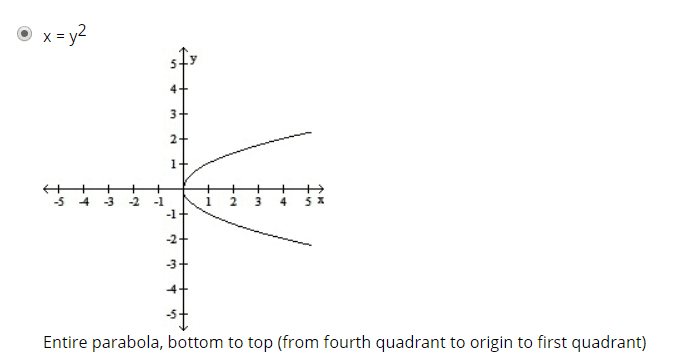# Graph the Cartesian equation: x=4t^2, y=2t

• Fatima Hasan
Also, the direction of motion is not specified in the given equations, but we can assume it is in the positive x-direction. Therefore, in summary, the given parametric equations represent a parabola with the equation ##y^2 = x## in the xy-plane. The portion of the graph traced by the particle is the parabola itself and the direction of motion is in the positive x-direction.

## Homework Statement

Parametric equations and a parameter interval for the motion of a particle in the xy-plane are given. Identify the particle's path by finding a Cartesian equation for it. Graph the Cartesian equation. Indicate the portion of the graph traced by the particle and the direction of motion.

(1)##x = 4t^2##
(2) ##y = 2t## , ## - \infty ≤ t ≤ \infty##

-

## The Attempt at a Solution

Square the second equation :
##y^2 = 4 t^2 ## (3)
Subtract (3) - (1):
##y^2 - x = 0 ##
##y^2 = x##
This equation forms a parabola .
When ##t = - 1 ## , ##x=4## and ##y=-2##
When ## t = 1 ## , ##x = 4## and ##y=2 ##
From bottom to top.#### Attachments

Last edited by a moderator:
Fatima Hasan said:

## Homework Statement

Parametric equations and a parameter interval for the motion of a particle in the xy-plane are given. Identify the particle's path by finding a Cartesian equation for it. Graph the Cartesian equation. Indicate the portion of the graph traced by the particle and the direction of motion.

(1)##x = 4t^2##
(2) ##y = 2t## , ## - \infty ≤ t ≤ \infty##

-

## The Attempt at a Solution

Square the second equation :
##y^2 = 4 t^2 ## (3)
Subtract (3) - (1):
##y^2 - x = 0 ##
##y^2 = x##
This equation forms a parabola .
When ##t = - 1 ## , ##x=4## and ##y=-2##
When ## t = 1 ## , ##x = 4## and ##y=2 ##
From bottom to top.
View attachment 231783
•